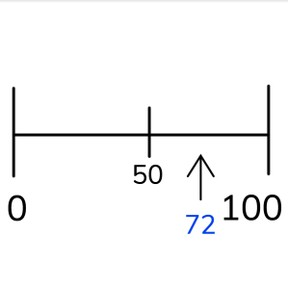Approximate placement of numbers to 100 on the number line

# Approximate placement of numbers to 100 on the number line

Approximate placement of numbers to 100 on the number line8,000 schools use Gynzy92,000 teachers use Gynzy1,600,000 students use Gynzy

## General

Students learn to approximate placement of numbers to 100 on a blank number line with only 0 and 100 visible.

-1.NBT.B.2

## Relevance

Discuss with students that it is important to know the approximate placement of numbers on a number line. If you know the approximate placement, it will help you determine which numbers are higher and lower than your given number.

## Introduction

Ask students to determine where the numbers from 0 to 100 belong on the number line. Ask them to drag the tens to their places on the number line.

## Development

Show students a number line with marks and without marks and ask them to explain the differences that they see. Discuss that on the first number line you can place a number exactly where it belongs, whereas on the second you place it approximately where it belongs. Discuss the term "approximately" and explain that it means that you make a best guess, but don't know exactly. Tell students that when you place numbers on an empty number line that you first must find the middle. The number that is in the middle between 0 and 100 is 50. Place a number on the number line by finding the middle, and then deciding if the number is bigger or smaller than 50. Then you need to determine the neighboring tens numbers of the given number and place the number. The first number to place is under 25. The next number is 85. With 85, teach students a second way to determine approximate placement. First find your middle(50). Then, find the middle between 0 and 50(25), and 50 and 100(75). 85 is between 75 and 100 and you place the number closer to 75 than to 100 on the number line. Students practice placing more numbers on the number line. Have students explain their reasoning and logical steps to reach their placement. The first number is clearly just below the middle. The second number is higher than 50 and between 75 and 100. Next students are asked to determine which number could be represented with the question mark on a number line. The number is below the middle and between 0 and 25, closer to 25. Check their answers and check to see if the answer falls within the given margin.

Check that students understand approximate placement of numbers to 100 by asking the following questions:
- Which steps can you take to help determine approximate placement of a number?
- If you have a number line from 0 to 100, which number(s) are helpful to place first?
- How do you approximately place a number on the number line? (find the middle, neighboring tens numbers, placement)

## Guided practice

Students first practice placing numbers on an empty number line, where the middle is given and students choose from multiple choice. Ask them how they decided on an answer, and ask them to explain their steps. Summarize the steps by indicating that the number is between 0 and 50 and 0 and 10, so the answer is 5. Next they are asked to approximate placement of a number. Have students write this down. Have them share the steps they took to figure out the approximate placement. Finally they need to determine the indicated number. Tell the thinking steps of discovering the number is between 50 and 100, and between 75 and 100.

## Closing

Discuss with students that it is useful to know approximate placement of numbers to 100 on the number line, because it helps to determine when numbers are higher or lower. Check that students know the steps of approximate placement on the number line- finding the middle (50) and deciding if the given number is higher or lower. Then determining the neighboring tens numbers or if the number is between 0 and 25, 25 and 50, 50 and 75, or 75 and 100. Then they place the number. Ask students to explain and decide where the numbers 45 and 65 should approximately be on the number line.

## Teaching tips

Students who have difficulty can be supported by making and using a filled in number line to 100. Walk through the steps of making the number line with the student, tens, middle point, and end points. Also show the steps of approximate placement using the completed number line.

## Instruction materials

Number cards from 0 to 100

### The online teaching platform for interactive whiteboards and displays in schools

• Save time building lessons

• Manage the classroom more efficiently

• Increase student engagement